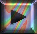Search:

# The determination of the charge and mass of the electron

## Robert A. MillikanThomson's experiment revealed the ratio of mass and charge $e$ of the electron: $r$ $=$ $\frac{m_{e^-}}{e}$ It was impossible so far to know these two physical parameter separately.

## The experiment

Millikan imagined a genial experiment to detect the charge of the electron:Small drops of oils are formed by a sprayer, and some of them attach themselves to electrons that have been produced by the action of xrays on molecules. The experimenter measures first the rate at which a drop falls in the earth's gravitational field. By charging the plates, drops carrying one additional electron fall with a different speed and so the charge of this electron can be calculated.

$e = -1,6 \cdot 10^{-19} C$

The charge $e$ of one electron is called elementary negative charge. A simple calculation gives then the electron mass: $m_{e^-}$ $=$ $r \cdot e$

$m_{e^-}$ $=$ $9,1 \cdot 10^{-31} kg$

More